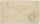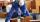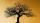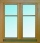Garden

Father dig up the garden in 9 hours. Son in 13 hours. How many hours take dig up the garden together?

Result

x =  5.318 h

Solution:

x/9+x/13 = 1

22x = 117

x = 11722 ≈ 5.318182

Calculated by our simple equation calculator.

Leave us a comment of example and its solution (i.e. if it is still somewhat unclear...):Be the first to comment!To solve this example are needed these knowledge from mathematics:

Looking for help with calculating harmonic mean? Looking for a statistical calculator? Need help calculate sum, simplify or multiply fractions? Try our fraction calculator. Do you have a linear equation or system of equations and looking for its solution? Or do you have quadratic equation?

Next similar examples:

1. NovakNovak needed to dig up three of the same pit in the garden. The first pit dug father alone for 15 hours. His second dig son helped him and it did that in six hours. The third pit dug son himself. How long it took him?
2. WorkersTwo workers will construct the stage at the festival in 54 hours. Organizers need to shorten the assembly time by 42 hours. How many workers must the assembly company send?
3. AuntAnnie aunt is 35 years old, Annie is 14 years. For how many years will aunt have twice as many years as Annie?
4. Bricklayers8 bricklayers build a house for 630 days. How many bricklayers have to add after 150 days, so then the whole building completed in (next) 320 days?
5. Orchard3/5 of the trees in the orchard are apples, 1/3 are cherries and the remaining five trees are pear. How many trees are in the orchard?
6. Workers 9If two workers fix two windows in an hour how many windows can 18 workers fix in the same two windows?
7. Hectoliters of waterThe pool has a total of 126 hectoliters of water. The first pump draws 2.1 liters of water per second. A second pump pumps 3.5 liters of water per second. How long will it take both pumps to drain four-fifths of the water at the same time?
8. Find unknownFind unknown numerator: 4/8 + _/8 = 1
9. Find xSolve: if 2(x-1)=14, then x= (solve an equation with one unknown)
10. PlayingHow long have we trained on the pitch when we know that the warm-up took 10 minutes, we trained passes for one-third of the time and we played football half the time?
11. GivenGiven 2x =0.125 find the value of x
12. One-thirdA one-third of unknown number is equal to five times as great as the difference of the same unknown number and number 28. Determine the unknown number.
13. NumberI think the number. If I add to its third seven I get same as when to its quarter add 8. Which is the number?
14. ExpressionSolve for a specified variable: P=a+4b+3c, for a
15. HotelThe hotel has a p floors each floor has i rooms from which the third are single and the others are double. Represents the number of beds in hotel.
16. Simplify 2Simplify expression: 5ab-7+3ba-9
17. Fraction to decimalWrite the fraction 3/22 as a decimal.